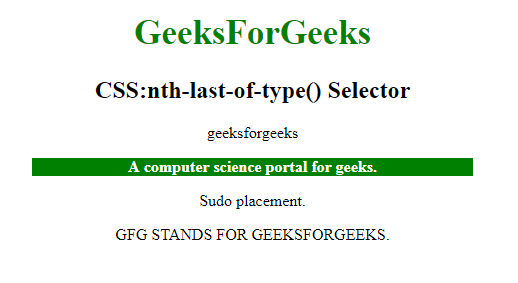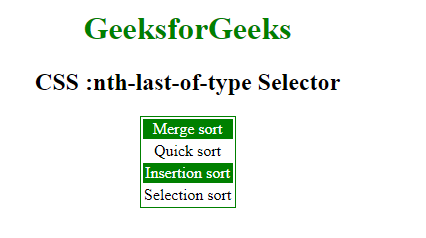Related Articles
CSS | :nth-last-of-type() Selector
• Last Updated : 15 Apr, 2019

The :nth-last-of-type() Selector in CSS is used to style only those elements which are the nth number of child of the parent element. The selector counting from the last-child element. A n could be a number, a keyword, or a formula.

Syntax:

```:nth-last-of-type(number) {
//css Property;
} ```

Where number is the argument that represents the pattern for matching elements counting from the end. It can be odd, even or in a functional notation.

• odd: It represents elements whose position is odd in a series: 1, 3, 5, etc., counting from the end.
• even: It represents the elements whose position is even in a series: 2, 4, 6, etc., counting from the end.
• functional notation (): It represents elements whose position of siblings matches the pattern An+B, for every positive integer or zero value of n. The index of the first element, counting from the end, is 1.

Example-1:

 ` ` `<``html``> ` ` `  `<``head``> ` `    ``<``style``> ` `        ``h1 { ` `            ``color: green; ` `            ``font-size: 35px; ` `        ``} ` `         `  `        ``p:nth-last-of-type(3) { ` `            ``background: green; ` `            ``color: white; ` `            ``font-weight: bold; ` `            ``width: 70%; ` `        ``} ` `    `` ` ` ` ` `  `<``body``> ` `    ``<``center``> ` `        ``<``h1``>GeeksForGeeks ` `        ``<``h2``>CSS:nth-last-of-type() Selector ` `        ``<``p` `class``=``"geeksFORGEEKS"``>geeksforgeeks ` `            ``<``p` `class``=``"forgeeks"``>A computer science portal for geeks. ` `                ``<``p` `class``=``"geeks"``>Sudo placement. ` `                ``<``p` `class``=``"SUDO"``>GFG STANDS FOR GEEKSFORGEEKS. ` ` `  ` ` ` `  `                 `

Output :Example-2: In this example every even element is selected according to formula 2n counting from the end.

 ` ` `<``html``> ` ` `  `<``head``> ` `    ``<``title``>CSS :nth-last-of-type Selector ` `    ``<``style``> ` `        ``table { ` `            ``border: 1px solid green; ` `            ``margin: auto; ` `        ``} ` `        ``/* Selects the last three element */ ` `         `  `        ``tr:nth-last-of-type(2n) { ` `            ``background-color: green; ` `            ``color: white; ` `        ``} ` `    `` ` ` ` ` `  `<``body` `style``=``"text-align:center;"``> ` ` `  `    ``<``h1` `style``=``"color:green;"``>  ` `            ``GeeksforGeeks  ` `        `` ` ` `  `    ``<``h2``>  ` `            ``CSS :nth-last-of-type Selector  ` `        `` ` ` `  `    ``<``table``> ` `        ``<``tbody``> ` `            ``<``tr``> ` `                ``<``td``>Merge sort ` `            `` ` `            ``<``tr``> ` `                ``<``td``>Quick sort ` `            `` ` `            ``<``tr``> ` `                ``<``td``>Insertion sort ` `            `` ` `            ``<``tr``> ` `                ``<``td``>Selection sort ` `            `` ` `        `` ` `    `` ` ` `  ` ` ` `  ` `

Output :Supported Browsers: The browser supported by :nth-last-of-type() Selector are listed below:

• Apple Safari